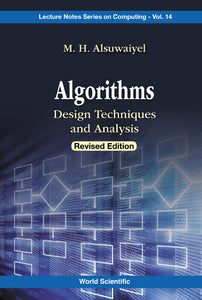# Algorithms: Design Techniques And Analysis (Revised Edition)

M H Alsuwaiyel

Format: Print Book

ISBN: 9789814723640

• SGD 264.29
Unit price per
Tax included.

Problem solving is an essential part of every scientific discipline. It has two components: (1) problem identification and formulation, and (2) the solution to the formulated problem. One can solve a problem on its own using ad hoc techniques or by following techniques that have produced efficient solutions to similar problems. This requires the understanding of various algorithm design techniques, how and when to use them to formulate solutions, and the context appropriate for each of them.Algorithms: Design Techniques and Analysis advocates the study of algorithm design by presenting the most useful techniques and illustrating them with numerous examples — emphasizing on design techniques in problem solving rather than algorithms topics like searching and sorting. Algorithmic analysis in connection with example algorithms are explored in detail. Each technique or strategy is covered in its own chapter through numerous examples of problems and their algorithms.Readers will be equipped with problem solving tools needed in advanced courses or research in science and engineering.

Format: Hardcover
No of Pages: 572
Imprint: World Scientific
Publication date: 20160216
Series: Lecture Notes Series On Computing